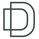TradingView3432 views
Maximum Drawdown and Maximum Relative Drawdown% calculating functions.

I needed a way to calculate the maxDD% of a serie of datas from an array (the different values of my balance account). I didn't find any builtin pinescript way to do it, so here it is.

There are 2 algorithms to calculate maxDD and relative maxDD%, one non optimized needs n*(n - 1)/2 comparisons for a collection of n datas, the other one only needs n-1 comparisons.

In the example we calculate the maxDDs of the last 10 close values.

There a 2 functions : "maximum_relative_drawdown" and "maximum_dradown" (and "optimized_maximum_relative_drawdown" and "optimized_maximum_drawdown") with names speaking for themselves.

Input : an array of floats of arbitrary size (the values we want the DD of)
Output : an array of 4 values

I added the iteration number just for fun.

Basically my script is the implementation of these 2 algos I found on the net :

var peak = 0;
var n = prices.length
for (var i = 1; i < n; i++){
dif = prices - prices;
peak = dif < 0 ? i : peak;
maxDrawdown = maxDrawdown > dif ? maxDrawdown : dif;
}

var n = prices.length
for (var i = 0; i < n; i++){
for (var j = i + 1; j < n; j++){
dif = prices - prices;
maxDrawdown = maxDrawdown > dif ? maxDrawdown : dif;
}
}

Feel free to use it.

@version=4
Open-source script

In true TradingView spirit, the author of this script has published it open-source, so traders can understand and verify it. Cheers to the author! You may use it for free, but reuse of this code in a publication is governed by House Rules. You can favorite it to use it on a chart.

Want to use this script on a chart?

## CommentsI forgot to explain the output of the functions : it's an array of 4 values : maxDD (or maxDD%) peak trough and iterations.
That's why I calculate the maxDD this way :

result = array.new_float(4)
result := maximum_relative_drawdown(array_of_values)

And I access the value like this :

maxdd := array.get(result, 0)

If you need the peak or the trough
peak := array.get(result, 1)
trough := array.get(result, 2)
Replysickojacko
@sickojacko, Thanks for the explanation on this part of the code. Amazing work, thanks for sharing it.
ReplyThis publication is now featured in our Editors' Picks: https://www.tradingview.com/scripts/editors-picks/ .
In the name of all TradingViewers, thank you for your valuable contribution to the community, and congrats!
Reply